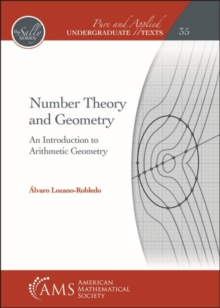Supporting your high street Find out how »
• My Account# Number Theory and Geometry : An Introduction to Arithmetic Geometry Hardback

## Part of the Pure and Applied Undergraduate Texts series

#### Description

Geometry and the theory of numbers are as old as some of the oldest historical records of humanity.

Ever since antiquity, mathematicians have discovered many beautiful interactions between the two subjects and recorded them in such classical texts as Euclid's Elements and Diophantus's Arithmetica.

Nowadays, the field of mathematics that studies the interactions between number theory and algebraic geometry is known as arithmetic geometry.

This book is an introduction to number theory and arithmetic geometry, and the goal of the text is to use geometry as the motivation to prove the main theorems in the book.

For example, the fundamental theorem of arithmetic is a consequence of the tools we develop in order to find all the integral points on a line in the plane.

Similarly, Gauss's law of quadratic reciprocity and the theory of continued fractions naturally arise when we attempt to determine the integral points on a curve in the plane given by a quadratic polynomial equation.

After an introduction to the theory of diophantine equations, the rest of the book is structured in three acts that correspond to the study of the integral and rational solutions of linear, quadratic, and cubic curves, respectively. This book describes many applications including modern applications in cryptography; it also presents some recent results in arithmetic geometry.

With many exercises, this book can be used as a text for a first course in number theory or for a subsequent course on arithmetic (or diophantine) geometry at the junior-senior level.

#### Information

• Format: Hardback
• Pages: 488 pages
• Publisher: American Mathematical Society
• Publication Date:
• Category: Number theory
• ISBN: 9781470450168

£107.00

on all orders

###### Pick up orders

from local bookshops

£41.50

£41.29

£97.95

£96.59

£80.95

£80.25

£80.95

£80.25

£80.95

£80.25